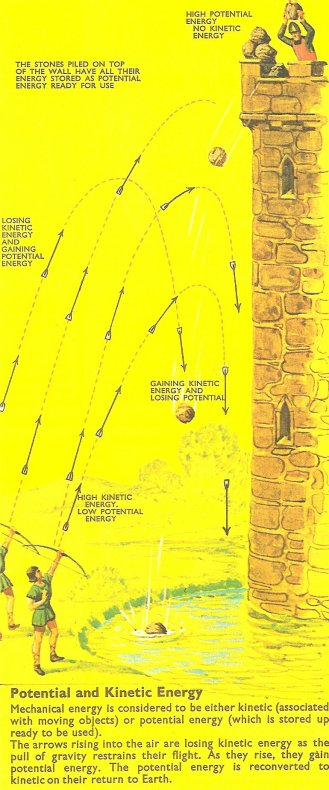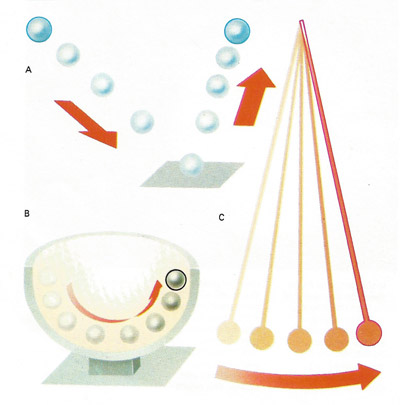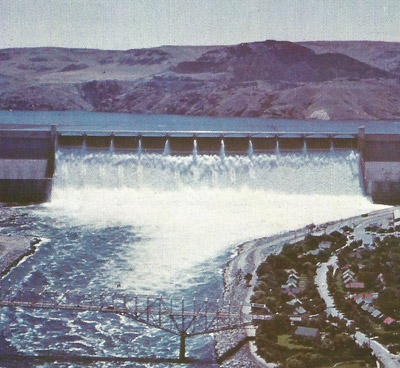A# potential energyFig 1. Potential and kinetic energy.Fig 2. Change from potential to kietic energy. A dam holds water at a height and when the water is released its potential energy changes to kinetic energy that can be converted into useful electrical energy.Fig 3. A dam holds water at a height and when the water is released its potential energy changes to kinetic energy that can be converted into useful electrical energy by water turbine generators.

Potential energy is stored energy or energy possessed by an object due to its position, shape, or state. The most familiar example of potential energy is that of an object raised to a certain height above Earth's surface. The object has potential energy because of its position in Earth's gravitational field. It has more potential energy than when on the surface by the amount mgh, where m is the object's mass, g is the acceleration due to gravity, and h is the height above the surface. (This relationship is true provided that g is constant, which is approximately true if h is small.)

In general, any object that occupies a position in a force field has potential energy. The force field may be gravitational, electrical, or nuclear. In addition, there is elastic potential energy (the stored energy of an object, such as a bow, that is deformed under tension or compression), chemical potential energy, and thermal potential energy.

In a coiled spring, the potential energy is proportional to the square of the compression or extension. A copper sphere insulated from electrical leakage can be charged with static electricity (stationary electrons) and the electrical potential energy of the sphere is determined by the amount of static electric charge and the associated voltage.

## Change from potential to kinetic energy

See Fig 2. The change from potential energy, due to an object's position, to kinetic energy, due to its movement, and back again occurs with a bouncing ball (A), a ball rolling a hemispherical cup (B) and a swinging pendulum (C). Before a ball is dropped it possesses only potential energy. This is converted to kinetic energy as the ball falls and reaches a maximum at the moment of impact – when potential energy is zero. Potential energy is regained as the ball rises to become stationary again at the top of the bounce. The same sequence of transitions occur in the bob at the end of the pendulum and with the ball rolling in its cup.